# Appendix C: Implementation Requirements

### Contents

This appendix is normative.

## C.1 Introduction

The following are notes about implementation requirements corresponding to various features in the SVG language.

## C.2 Version control

The SVG user agent must verify the reference to the SVG DTD in the `<!DOCTYPE>` statement or the `xmlns` attribute to ensure that it identifies the DTD for a version of the SVG language which the SVG user agent supports. If the reference to the DTD is missing or it does not correspond to a version which the SVG user agent supports, then the SVG user agent must not attempt to process the given SVG document fragment. If the user environment allows it, the user agent must generate an appropriate error message with a suggested alternative processing option (e.g., installing an updated version of the user agent) if such an option exists.

In particular, SVG user agents must not attempt to process SVG document fragments whose `<!DOCTYPE>` statement or corresponding `xmlns` attribute references a DTD corresponding to a working draft version of the SVG specification. These document fragments need to be updated to the SVG Recommendation before they can be rendered by SVG user agents which support the SVG Recommendation. If the user environment allows it, the user agent must generate an appropriate error message indicating that the SVG document fragment is not a conforming SVG Document fragment.

## C.3 Forward and undefined references

SVG makes extensive use of URI references to other objects. For example, to fill a rectangle with a linear gradient, you define a 'linearGradient' element and give it an ID (e.g., <linearGradient id="MyGradient"...>, and then you can specify the rectangle as follows: <rect style="fill:url(#MyGradient)"...>.

In SVG, among the facilities that allow URI references are:

Forward references are disallowed. All references must be to elements which are either defined in a separate document or defined earlier in same document. References to elements in the same document can only be to elements which are direct children of a 'defs' element. (See Defining referenced and undrawn elements: the 'defs' element.).

Unless a given attribute or property has defined fallback behavior when a reference cannot be resolved (e.g., typically, when a list of alternative values is provided), invalid references are treated as errors (see Error Processing). For example, if there is no element with ID "BogusReference" in the current document, then fill="url(#BogusReference)" would represent an invalid reference and would be an error.

## C.4 Referenced objects are "pinned" to their own coordinate systems

Except in the cases where value userSpaceOnUse or objectBoundingBox is assigned to:

when a graphical object is referenced by another graphical object, the referenced object does not change location, size or orientation. Thus, referenced graphical objects are "pinned" to the user coordinate system that is in place within its own hierarchy of ancestors and is not affected by the user coordinate system of the referencing object.

## C.5 Clamping values which are restricted to a particular range

Some numeric attribute and property values have restricted ranges, such as color component values. When out of range values are provided, but user agent shall defer any error checking until after presentation time, as composited actions might produce intermediate values which are out of range but final values which are within range.

Color values are not in error if they are out of range, even if final computations produce an out of range color value at presentation time. It is recommended that user agents clamp color values to the nearest color value (possibly determined by simple clipping) which the system can process as late as possible (e.g., presentation time), although it is acceptable for user agents to clamp color values as early as parse time. Thus, implementation dependencies might preclude consistent behavior across different systems when out of range color values are used.

Opacity values out of range are not in error and should be clamped to the range 0 to 1 at the time which opacity values have to be processed (e.g., at presentation time or when it is necessary to perform intermediate filter effect calculations).

## C.6 'path' element implementation notes

A conforming SVG user agent must implement path rendering as follows:

• Error handling:
• The general rule for error handling in path data is that the SVG user agent shall render a 'path' element up to (but not including) the path command containing the first error in the path data specification. This will provide a visual clue to the user/developer about where the error might be in the path data specification. This rule will greatly discourage generation of invalid SVG path data.
• If a path data command contains an incorrect set of parameters, then the given path data command is rendered up to and including the last correctly defined path segment, even if that path segment is a sub-component of a compound path data command, such as a "lineto" with several pairs of coordinates. For example, for the path data string "M 10,10 L 20,20,30", there is an odd number of parameters for the "L" command, which requires an even number of parameters. The user agent is required to draw the line from (10,10) to (20,20) and then perform error reporting since "L 20 20" is the last correctly defined segment of the pat data specification.
• Wherever possible, all SVG user agents shall report all errors to the user.
• Markers, directionality and zero-length path segments:
• If markers are specified, then a marker is drawn on every applicable vertex, even if the given vertex is the end point of a zero-length path segment and even if "moveto" commands follow each other.
• Certain line-capping and line-joining situations and markers require that a path segment have directionality at its start and end points. Zero-length path segments have no directionality. In these cases, the following algorithm is used to establish directionality: to determine the directionality of the start point of a zero-length path segment, go backwards in the path data specification within the current subpath until you find a segment which has directionality at its end point (e.g., a path segment with non-zero length) and use its ending direction; otherwise, temporarily consider the start point to lack directionality. Similarly, to determine the directionality of the end point of a zero-length path segment, go forwards in the path data specification within the current subpath until you find a segment which has directionality at its start point (e.g., a path segment with non-zero length) and use its starting direction; otherwise, temporarily consider the end point to lack directionality. If the start point has directionality but the end point doesn't, then the end point uses the start point's directionality. If the end point has directionality but the start point doesn't, then the start point uses the end point's directionality. Otherwise, set the directionality for the path segment's start and end points to align with the positive X-axis in user space.
• If 'stroke-linecap' is set to butt and the given path segment has zero length, do not draw the linecap for that segment; however, do draw the linecap for zero-length path segments when 'stroke-linecap' is set to either round or square. (This allows round and square dots to be drawn on the canvas.)
• The S/s commands indicate that the first control point of the given cubic bezier segment is calculated by reflecting the previous path segments second control point relative to the current point. The exact math is as follows. If the current point is (curx, cury) and the second control point of the previous path segment is (oldx2, oldy2), then the reflected point (i.e., (newx1, newy1), the first control point of the current path segment) is:
```
(newx1, newy1) = (curx - (oldx2 - curx), cury - (oldy2 - cury))
= (2*curx - oldx2, 2*cury - oldy2)
```
• A non-positive radius value is an error.
• Unrecognized contents within a path data stream (i.e., contents that are not part of the path data grammar) is an error.

## C.7 Elliptical arc implementation notes

### C.7.1 Elliptical arc syntax

An elliptical arc is a particular path command.  As such, it is described by the following parameters in order:

(x1, y1) are the absolute coordinates of the current point on the path, obtained from the last two parameters of the previous path command.

rX and rY are the radii of the ellipse (also known as its semi-major and semi-minor axes).is the angle from the x-axis of the current coordinate system to the x-axis of the ellipse.

fA is the large arc flag, and is 0 if an arc spanning less than or equal to 180 degrees is chosen, or 1 if an arc spanning greater than 180 degrees is chosen.

fS is the sweep flag, and is 0 if the line joining center to arc sweeps through decreasing angles, or 1 if it sweeps through increasing angles.

(x2, y2) are the absolute coordinates of the final point of the arc.

This parameterization of elliptical arcs will be referred to as endpoint parameterization.  One of the advantages of endpoint parameterization is that it permits a consistent path syntax in which all path commands end in the coordinates of the new "current point".  The following notes give rules and formulae to help implementers deal with endpoint parameterization.

### C.7.2 Out-of-range parameters

Arbitrary numerical values are permitted for all elliptical arc parameters, but where these values are invalid or out of range, an implementation must make sense of them as follows:

If the endpoints (x1, y1) and (x2, y2) are identical, then this is equivalent to omitting the elliptical arc segment entirely.

If rX = 0 or rY = 0 then this arc is treated as a straight line segment (a "lineto") joining the endpoints.

If rX or rY have negative signs, these are dropped (the absolute value is used instead).

If rX , rY andare such that there is no solution (basically, the ellipse is not big enough to reach from (x1, y1) to (x2, y2)) then the ellipse is scaled up uniformly until there is exactly one solution (until the ellipse is just big enough).is taken mod 360 degrees.

Any nonzero value for either of the flags fAor fS is taken to mean the value 1.

This forgiving yet consistent treatment of out-of-range values ensures that

The inevitable approximations arising from computer arithmetic cannot cause a valid set of values written by one SVG implementation to be treated as invalid when read by another SVG implementation.  This would otherwise be a problem for common boundary cases such as a semicircular arc.

Continuous animations that cause parameters to pass through invalid values are not a problem.  The motion remains continuous.

### C.7.3 Parameterization alternatives

An arbitrary point (x, y) on the elliptical arc can be described by the 2-dimensional matrix equation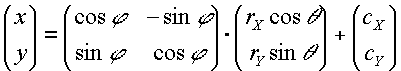(C.7.3.1)

(cX, cY) are the coordinates of the center of the ellipse.

rX and rY are the radii of the ellipse (also known as its semi-major and semi-minor axes).is the angle from the x-axis of the current coordinate system to the x-axis of the ellipse.ranges from: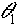which is the start angle of the elliptical arc prior to the stretch and rotate operations.which is the end angle of the elliptical arc prior to the stretch and rotate operations.which is the difference between these two angles.

If one thinks of an ellipse as a circle that has been stretched and then rotated, then,andare the start angle, end angle and sweep angle, respectively of the arc prior to the stretch and rotate operations.  This leads to an alternate parameterization which is common among graphics APIs, which will be referred to as center parameterization.  In the next sections, formulas are given for mapping in both directions between center parameterization and endpoint parameterization.

### C.7.4 Conversion from center to endpoint parameterization

Given: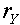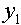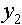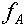Here are the formulas: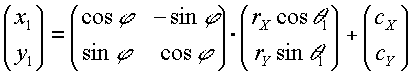(C.7.4.1)(C.7.4.2)(C.7.4.3)(C.7.4.4)

### C.7.5 Conversion from endpoint to center parameterization

Given:The equations simplify after a translation which places the origin at the midpoint of the line joining (x1, y1) to (x2, y2), followed by a rotation to line up the coordinate axes with the axes of the ellipse.  All transformed coordinates will be written with primes.  They are computed as intermediate values on the way toward finding the required center parameterization variables.  This procedure consists of the following steps:

Step 1: Compute (x1', y1') according to the formula(C.7.5.1)

Step 2: Compute (cX ', cY ') according to the formula(C.7.5.2) where the + sign is chosen if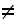and the - sign is chosen ifStep 3: Compute (cX, cY) from (cX ', cY ')(C.7.5.3)

 Step 4: ComputeandIn general, the angle between two vectors (uX, uY) and (vX, vY) can be computed as(C.7.5.4) where the ± sign appearing here is the sign of uX vY - uY vX

 This angle function can be used to expressandas follows: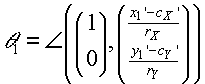(C.7.5.5)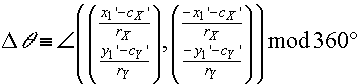(C.7.5.6)

 whereis fixed in the range -360° << 360° such that:
 if0, then< 0,
 else if1, then> 0.
 In other words, if fS = 0 and the right side of (C.7.5.6) is > 0, then subtract 360°, whereas if fS = 1 and the right side of (C.7.5.6) is < 0, then add 360°.  In all other cases leave it as is.

### C.7.6 Correction of out-of-range radii

This section formalizes the adjustments to out-of-range rX and rY mentioned in C.7.2. Algorithmically these adjustments consist of the following steps:

Step 1: Ensure radii are non-zero

If rX = 0 or rY = 0, then treat this as a straight line from (x1, y1) to (x2, y2) and stop.  Otherwise,

Step 2: Ensure radii are positive

Take the absolute value of rX and rY:(C.7.6.1)

Step 3: Ensure radii are large enough

Using the primed coordinate values of equation (C.7.5.1), compute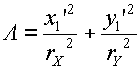(C.7.6.2)

If the result of the above equation is less than or equal to 1, then no further change need be made to rX and rY.  If the result of the above equation is greater than 1, then make the replacements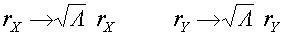(C.7.6.3)

Step 4: Proceed with computations

Proceed with the remaining elliptical arc computations, such as those in section C.7.5.  Note: As a consequence of the radii corrections in this section, equation (C.7.5.2) for the center of the ellipse always has at least one solution (i.e. the radicand is never negative).  In the case that the radii are scaled up using equation (C.7.6.3), the radicand of (C.7.5.2) is zero and there is exactly one solution for the center of the ellipse.

## C.8 Text selection implementation notes

The following implementation notes describe the algorithm for deciding which characters are selected during a text selection operation.

The text selection operation determines the start selection character(s) and start selection subregion and the end selection character(s) and end selection subregion. To determine the start selection character(s), the SVG user agent determines which rendered glyph received the initial select event (e.g., the initial mouse down event) and which character(s) corresponds to the given glyph (note that for ligatures, a single glyph represents multiple characters). For all of the glyphs used to render the given character(s), determine start selection subregion depending on whether the selection/pointer event occurred in the top/left, top/right, bottom/left or bottom/right subregion of the character cell area that encompasses all of the glyphs used to render the given character(s).

The end character(s) and the relevant end character(s) subregion are determined using a similar method, except use the pointer location at the end of the select operation (e.g., when the user releases the given mouse button).

For systems which support pointer devices such as a mouse, the user agent is required to provide a mechanism for selecting text even when the given text has associated event handlers or links, which might block text selection due to event processing precedence rules (see Pointer events). One implementation option for platforms which support a pointer device such as a mouse, the user agent may provide for a small additional region around character cells which initiate text selection operations but do not initiate event handlers or links.

For horizontal text (i.e., when the baseline of the glyph is parallel to the primary text advance direction):

• If the start selection subregion is either the top/left or bottom/left, then the selection starts between the start character(s) and the previous character in visual rendering order for the 'text' element. (If this is the first character in visual rendering order for the 'text' element, then the selection starts with this first character in visual rendering order. Note that the bi-directional algorithm might result in the selection being between two characters that are not contiguous in lexical order.)
• If the start selection subregion is either the top/right or bottom/right, then the selection starts between the start character(s) and the next character in visual rendering order for the 'text' element. (If this is the last character in visual rendering order for the 'text' element, then the selection starts with this last character in visual rendering order. Note that the bi-directional algorithm might result in the selection being between two characters that are not contiguous in lexical order.)

For vertical text (i.e., when the baseline of the glyph is perpendicular to the primary text advance direction):

• If the start selection subregion is either the top/left or top/right, then the selection starts between the start character(s) and the previous character in visual rendering order for the 'text' element. (If this is the first character in visual rendering order for the 'text' element, then the selection starts with this first character in visual rendering order. Note that the bi-directional algorithm might result in the selection being between two characters that are not contiguous in lexical order.)
• If the start selection subregion is either the bottom/left or bottom/right, then the selection starts between the start character(s) and the next character in visual rendering order for the 'text' element. (If this is the last character in visual rendering order for the 'text' element, then the selection starts with this last character in visual rendering order. Note that the bi-directional algorithm might result in the selection being between two characters that are not contiguous in lexical order.)

When the user agent is implementing selection of bi-directional text in lexical order and the selection starts (or ends) between characters which are not contiguous in lexical order, then there might be multiple potential combinations of characters that can be considered part of the selection. The algorithms to choose among the combinations of potential selection options shall choose the selection option which most closely matches the text string's visual rendering order.